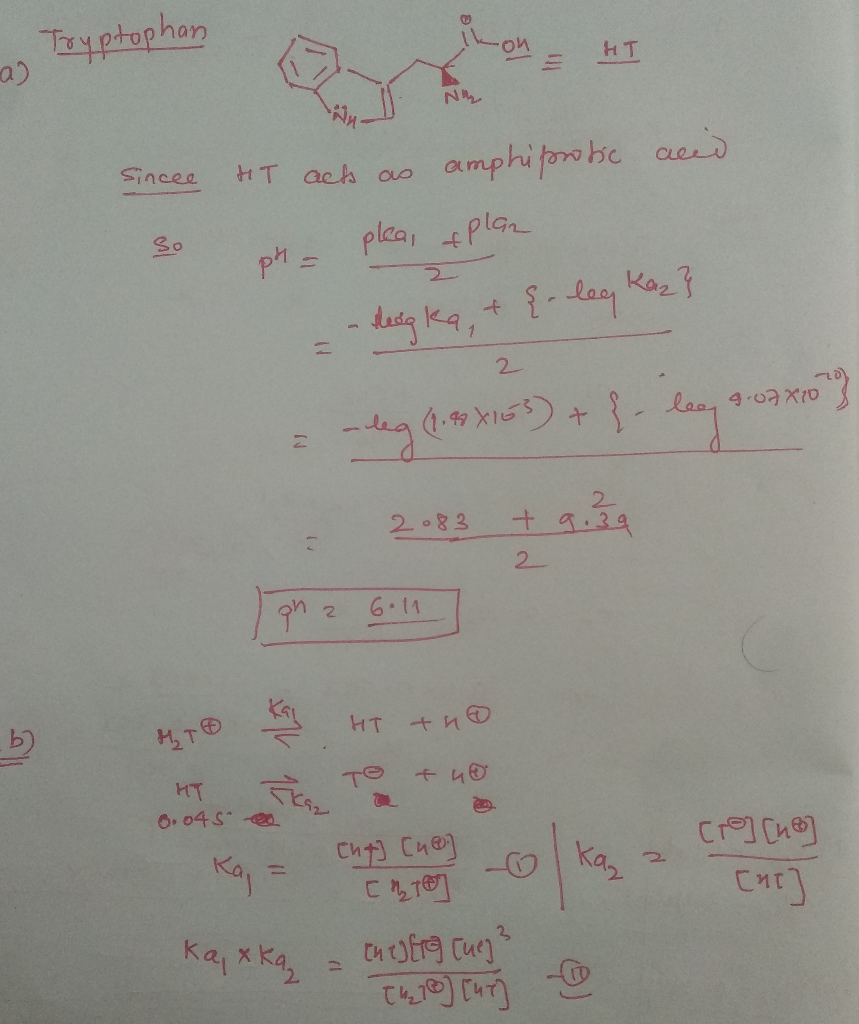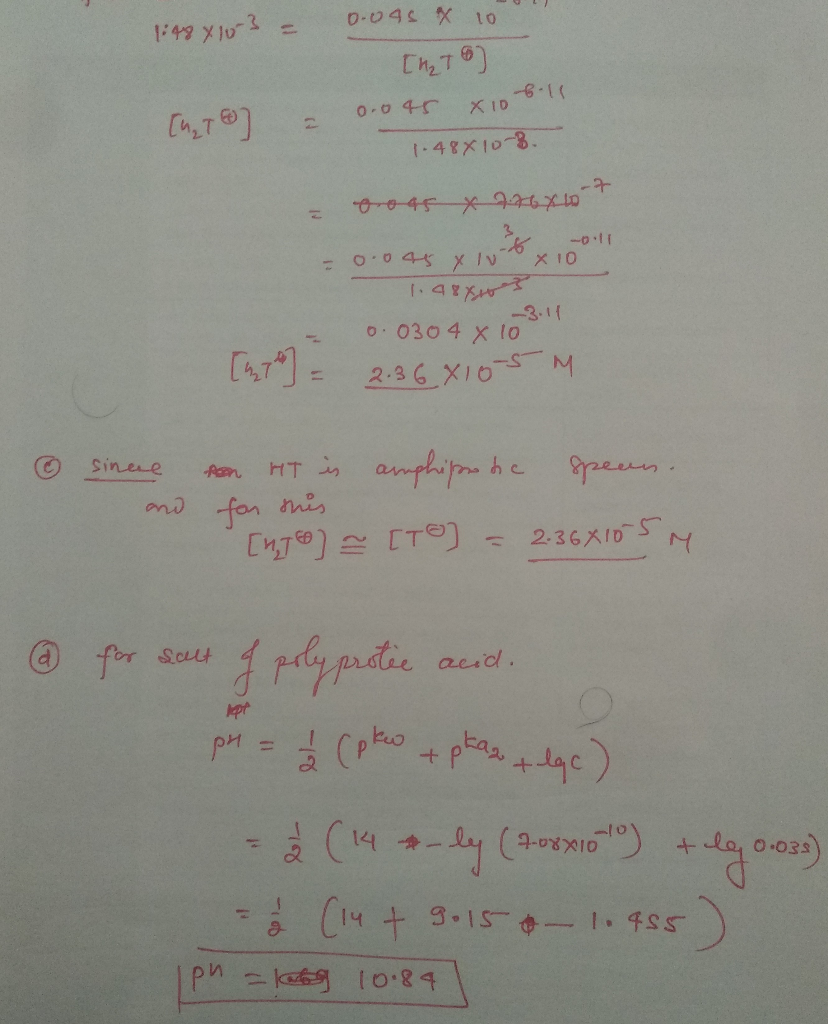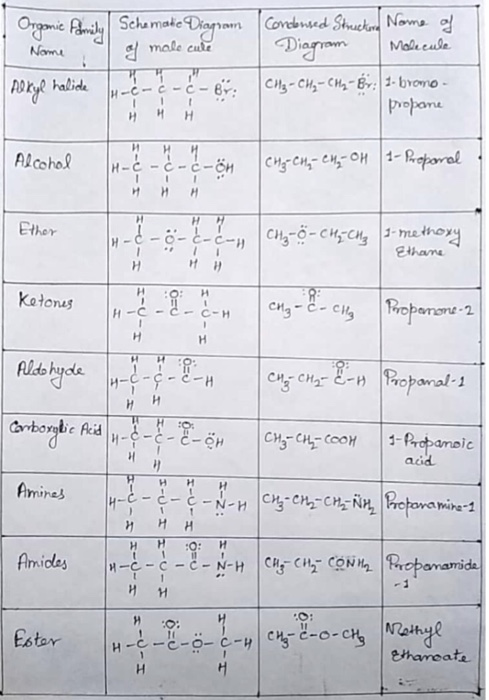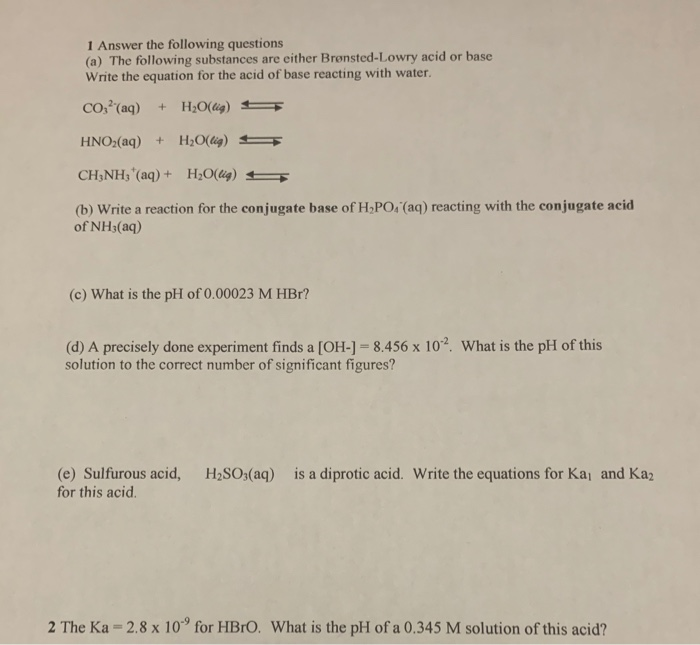# 1. Using the given information answer the following questions with corresponding answers. (a) Tryptophan is a...

1. Using the given information answer the following questions with corresponding answers.

(a)

Tryptophan is a diprotic acid (Ka1 = 1.48× 10-3 and Ka2 = 4.07× 10-10) that can have three different form in solution: H2T+, HT, and T-. What would be the pH of the solution containing 0.045M HT?

6.86

6.12

7.43

12.23

7.06

(b)

Tryptophan is a diprotic acid (Ka1 = 1.48× 10-3 and Ka2 = 4.07× 10-10) that can have three different form in solution: H2T+, HT, and T-. What would be the concentration of H2T+ in the solution of 0.045M HT?

4.2× 10-5 M

0.80× 10-5 M

5.1× 10-5 M

2.3× 10-5 M

3.7× 10-5 M

(c)

Tryptophan is a diprotic acid (Ka1 = 1.48× 10-3 and Ka2 = 4.07× 10-10) that can have three different form in solution: H2T+, HT, and T-. What would be the concentration of A- in the solution of 0.045M HT?

0.38× 10-5 M

3.3× 10-5 M

1.3× 10-5 M

2.4× 10-5 M

4.7× 10-5 M

(d)

Serine is a diprotic acid (Ka1 = 6.17× 10-3 and Ka2 = 7.08× 10-10) that can have three different forms in solution: H2S+, HS, and S- (Note: S is not sulfur in these formulas). What would be the pH of the solution containing 0.035M Na+S-?

9.88

10.23

10.85

9.02

3.15#### Earn Coin

Coins can be redeemed for fabulous gifts.

Similar Homework Help Questions
• ### Use the given information to answer the following questions with corresponding answers. (a) Consider the following disso...

Use the given information to answer the following questions with corresponding answers. (a) Consider the following dissociation reactions of glycine that can have three different forms in solution: H2G+, HG, or G-. : H2G+  ⟺   HG + H+ Ka1 = 4.6 × 10-3 HG  ⟺   G- + H+   Ka2 = 2.5 × 10-10 Determine the dissociation constant for G- + H2O  ⇔   HG + OH-. Group of answer choices 4.0 ×× 10-9 4.0 ×× 10-5 2.2 ×× 10-12 3.5 ×× 10-6 2.9 ××...

• ### 1. Using the given information answer the following questions with corresponding answers. (a) Serine is a diprotic acid...

1. Using the given information answer the following questions with corresponding answers. (a) Serine is a diprotic acid (Ka1 = 6.17 × 10-3 and Ka2 = 7.08 × 10-10) that can have three different forms in solution: H2S+, HS, and S- (Note: S is not sulfur in these formulas). What would be the concentration of H2S+ in the solution of 0.105M Na+S-? Group of answer choices 8.29 × 10-8 M 1.62 × 10-12 M 9.62 × 10-9 M 4.15 ×...

• ### 1 A) Which of the following pairs of solutions when mixed will form a buffer solution?...

1 A) Which of the following pairs of solutions when mixed will form a buffer solution? Group of answer choices H2SO4 and Ca(OH)2 NH3 and NH4Br NaNO3 and HNO3 KOH and KNO2 B)Phosphoric acid, H3PO4, is a triprotic acid with the following Ka values: Ka1 = 7.5 x 10-3; Ka2 = 6.2 x 10-8; Ka3 = 3.6 x 10-13 In a solution of 0.15 M H3PO4, which species will be most abundant? H3O+ H3PO4 HPO42- H2PO4-

• ### a) The consecutive equilibria: CO2(g) <=>CO2(aq) + H2O(l) <=>H2CO3(aq) <=>H+(aq) + HCO3-(aq), explain why: A. carbonated beverages are less acidic than pure water. B. carbonated beve...

a) The consecutive equilibria: CO2(g) <=>CO2(aq) + H2O(l) <=>H2CO3(aq) <=>H+(aq) + HCO3-(aq), explain why: A. carbonated beverages are less acidic than pure water. B. carbonated beverages contain measurably less water than the same volume of pure water. C. rainwater is slightly acidic b) A 1.0 M solution of a weak monoprotic acid with Ka = 1.0 10-6 would have a pH of: A. 1 B. 2 C. 3 D. 6 c) Ascorbic acid is a diprotic acid with Ka1 =...

• ### All of the following are acid-base conjugate pairs EXCEPT Group of answer choices H2O, H3O+ NH4+,...

All of the following are acid-base conjugate pairs EXCEPT Group of answer choices H2O, H3O+ NH4+, NH3 CH3COOH, CH3COO- H3O+, OH- HPO42-, PO43- The pH of a 1.86 M solution of a weak monoprotic acid, is 2.31. What is the Ka of the acid? Group of answer choices 4.7 x 10-5 4.1 x 10-7 3.4 x 105 2.4 x 10-4 1.3 x 10-5 You have 250.0 mL of a 0.56 M solution of NaCH3COO. How many milliliters of a 0.50...

• ### Adipic acid, HOOC(CH2)4COOH, is among the top 50 manufactured chemicals in the United States (nearly 1...

Adipic acid, HOOC(CH2)4COOH, is among the top 50 manufactured chemicals in the United States (nearly 1 million metric tons annually). Its chief use is in the manufacture of nylon. It is a diprotic acid having Ka1=3.9×10−5 and Ka2=3.9×10−6. A saturated solution of adipic acid is about 0.10 M HOOC(CH2)4COOH. A) Calculate the concentration of [H3O+][H3O+] in this solution. Express your answer to two significant figures and include the appropriate units. B) Calculate the concentration of [HOOC(CH2)4COO−][HOOC(CH2)4COO−] in this solution. Express...

• ### Using the information from the chart answer the following questions in full sentences.Using the information from the chart answer the following questions in full sentences. Mol ト1 Ether tonas ldo acid инн ihe-1 - CNH etharcate names of the compounds 2. Which of the compounds you built are structural isomers? Include the n and explain your choices. (4 marks) 3. Which of these compounds would have the highest solubility? Explain. (2 marks) 4. Select three of the molecules you have created above. Then draw and name a structural isomer that would still...

• ### LULTIPLE CHOICE. Choose the one alternative that best completes the statement or answers the question 1)...LULTIPLE CHOICE. Choose the one alternative that best completes the statement or answers the question 1) The compound BC13 can be described as a(n) 1) A) Lewis acid. B) Bronsted-Lowry base D) Arrhenius acid C) Lewis base. 2) What is the pH of a solution prepared by diluting 25.00 mL of 0.10 M HC1 with enough water to produce a total volume of 100.00 mL? A) 1.60 2) B) 2.00 9)3.20 D) 1.00 3) Which one of the following salts,...

• ### 1) One of these statements is incorrect. Which one? Group of answer choices Energy is the...

1) One of these statements is incorrect. Which one? Group of answer choices Energy is the capacity to do work or transfer heat. Exothermic processes transfer heat from the surroundings into the system. Endothermic processes have a positive ΔH value. Energy is neither created nor destroyed in chemical reactions. 2) Choose the compound from the set that can be classified as a salt. Group of answer choices sodium hydroxide (NaOH) acetic acid (CH3CO2H) glucose (C6H12O6) magnesium bromide (MgBr2) 3) Which...

• ### Hello! Can someone help me with these questions? Thank you! 1 Answer the following questions (a)...Hello! Can someone help me with these questions? Thank you! 1 Answer the following questions (a) The following substances are either Brønsted-Lowry acid or base Write the equation for the acid of base reacting with water. CO2?"(aq) + H2O(lig) - HNO3(aq) + H2O(lig) = CH3NH; (aq) + H2O(lig) = (b) Write a reaction for the conjugate base of H,PO, (aq) reacting with the conjugate acid of NH3(aq) (c) What is the pH of 0.00023 M HBr? (d) A precisely done...

Free Homework App